# Rotational Symmetry

Rotational Symmetry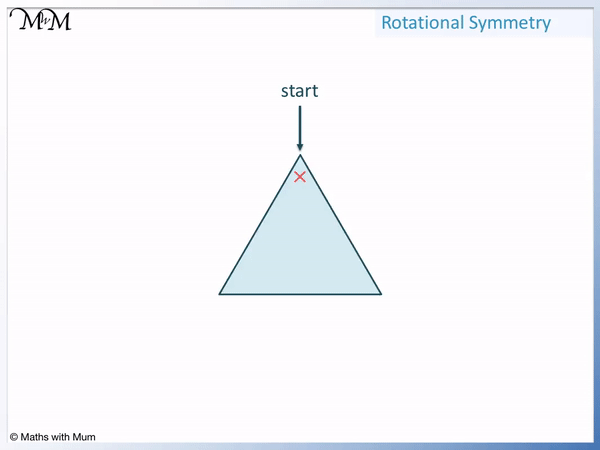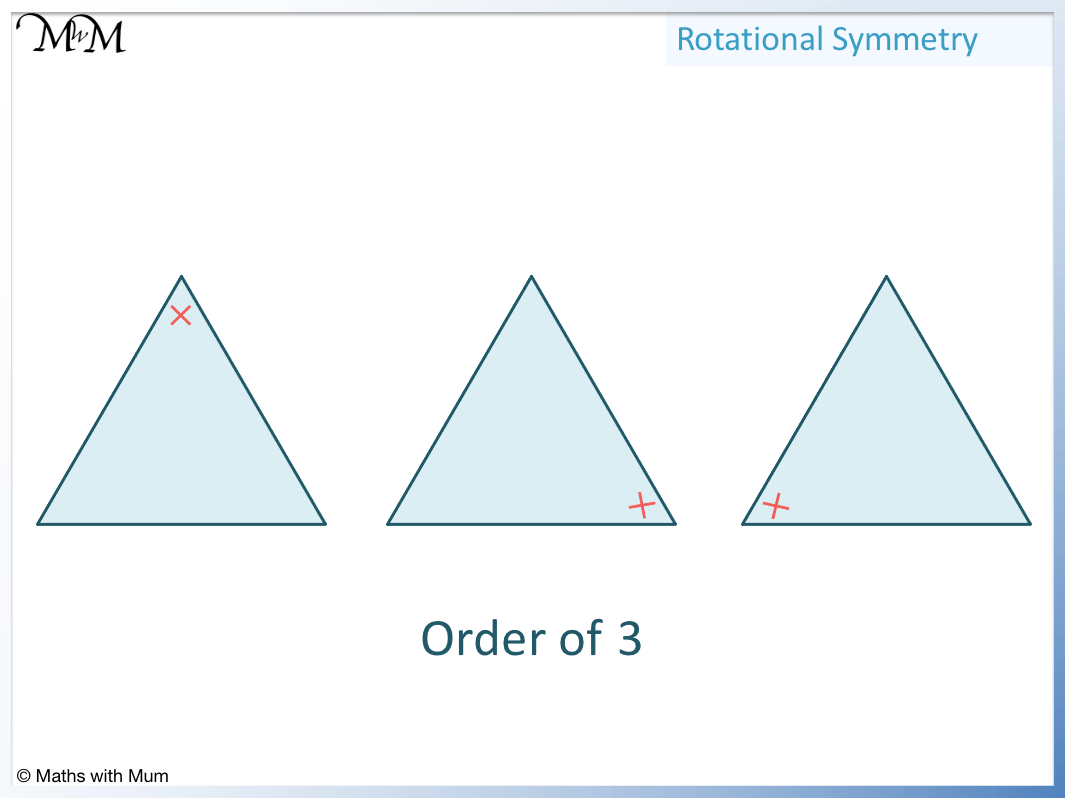• Rotational symmetry is when a shape is rotated and it looks exactly the same as before it was rotated.
• The order of rotational symmetry is the number of times a shape fits into its original outline when it is rotated a full turn.
• This equilateral triangle fits into its original outline 3 times and so it has a rotational symmetry order of 3.
• Marking one of the corners can help us to know when a full turn has been completed.

Rotate a shape a full turn and count the number of times the shape looks the same.

This number is called the order of rotational symmetry.• This shape has rotational symmetry order 2.
• This is because it fits into its original shape once when it is upside down and a second time when it completes a full turn.
• The angle of rotational symmetry is 180°. This is the first angle at which the shape fits inside its original outline.• This trapezoid only fits into its original outline once when it completes a full turn.
• We say that it has a rotational symmetry order of 1.
• Every shape will fit into its outline at least once. We cannot have a rotational symmetry order of zero.
• We say that this shape has no rotational symmetry.# Rotational Symmetry

## What is Rotational Symmetry?

Rotational symmetry is when an object has been rotated but it looks like it is in its original orientation. For example, a square will look the same when it is rotated a quarter turn. A shape does not have rotational symmetry if it does not look the same when rotated. For example, a kite has no rotational symmetry.

Below is a square showing rotational symmetry. We can see that a square can be rotated to 4 different positions and look the same.

The outside of the shape looks the same in the 4 different positions. When a shape demonstrates rotational symmetry, it looks like it has not been rotated at all.A kite is an example of a shape with no rotational symmetry. A shape with no rotational symmetry only fits into its original outline once. Shapes with no rotational symmetry have rotational symmetry order 1.We can see that the only time that the kite matches its outline is when it has not been rotated.

## What is the Order of Rotational Symmetry?

The order of rotational symmetry (or degree of rotational symmetry) is the number of times an object looks the same when it is rotated a full turn of 360°. The shape must look as though it has not rotated at all. For example, a rectangle has rotational symmetry of order 2. When it is turned 180° and 360° it looks the same as when it is at its starting position.

We can see that a rectangle always has rotational symmetry of order 2.

Here are two different rectangles with both rotational symmetries shown.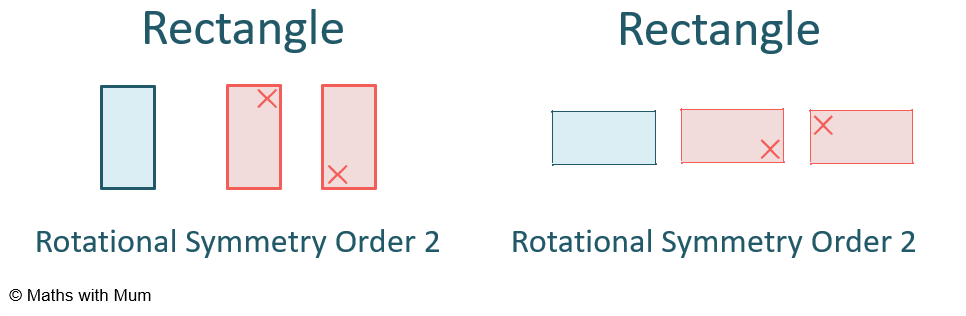We only count rotational symmetry over one full turn. Once the shape has returned to its original position, we stop. Otherwise we would continue to count onwards forever.

## How to Find the Order of Rotational Symmetry

To find the order of rotational symmetry:

1. Rotate the shape one full turn.
2. As it rotates, count the number of times the shape looks the same as before it was rotated.
3. This number is the order of rotational symmetry.

For example, find the order of rotational symmetry for this shape.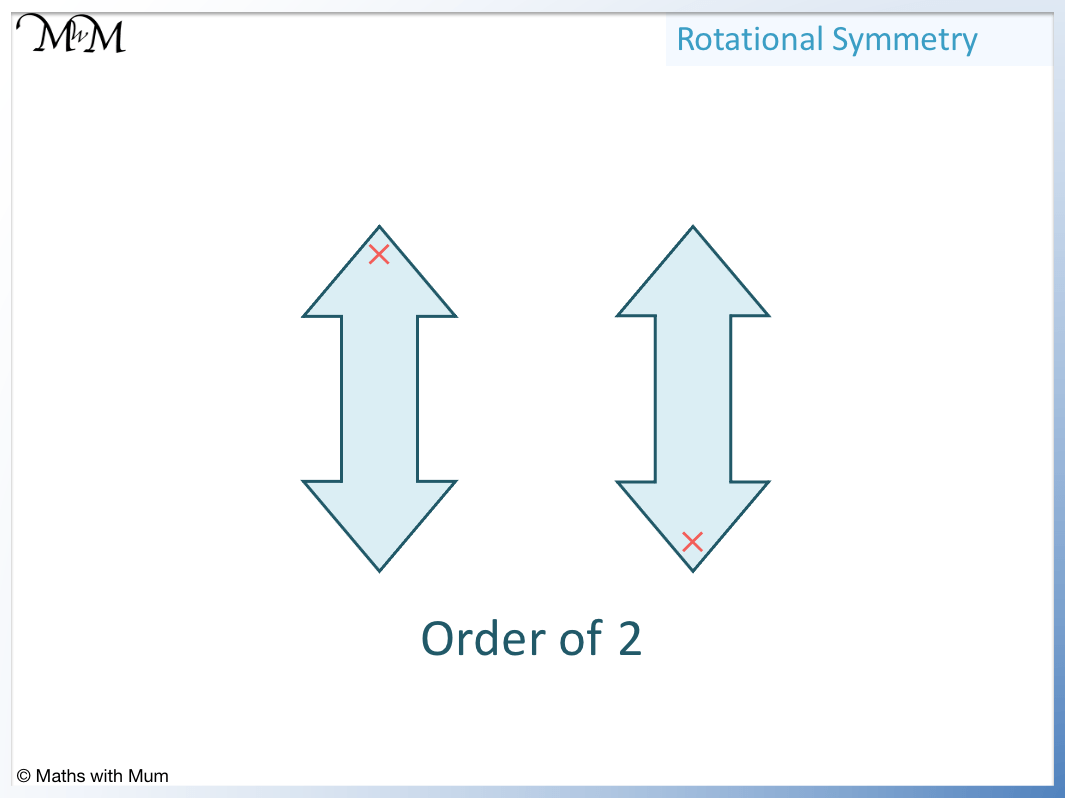Step 1 is to rotate the shape around one full turn.

Step 2 is to count the number of times the shape looks the same as before it was rotated. This is when it fits into its original outline.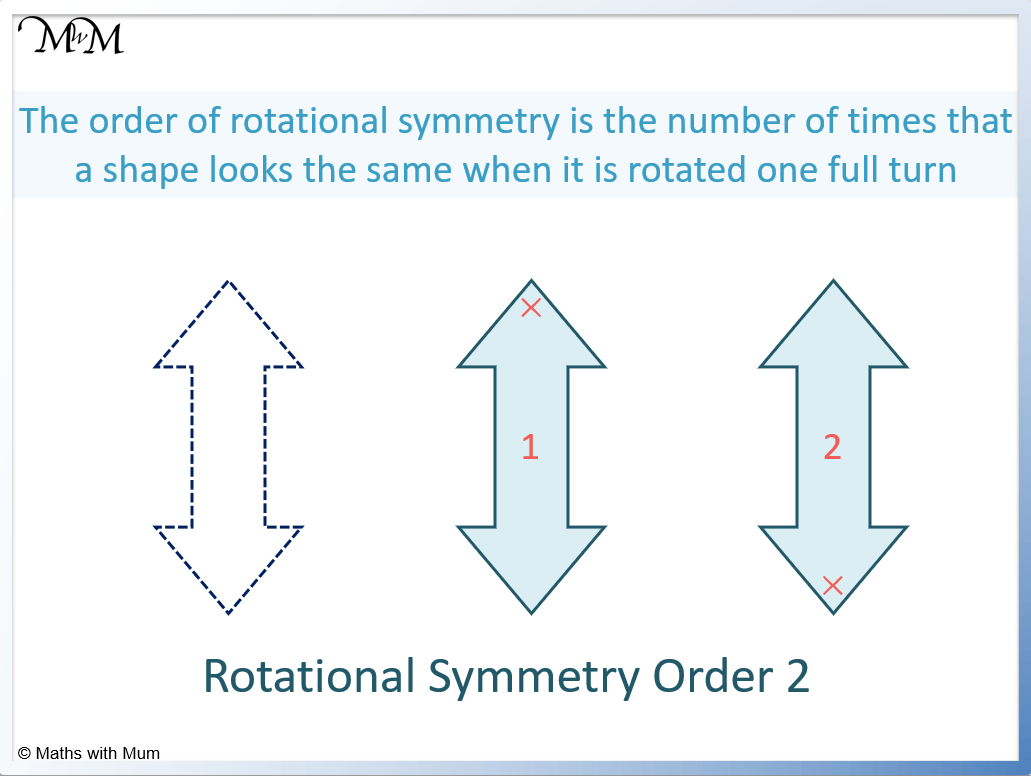The diagram above shows the two rotational symmetries of this shape.

The first symmetry is the position of the original shape and the second symmetry is the shape upside down after a rotation of 180°.

## Rotational Symmetry Using Tracing Paper

To find the order of rotational symmetry with tracing paper:

1. Place the tracing paper over the shape.
2. Draw around the outside of the shape.
3. Place your pencil in the centre of the shape and rotate the paper a full turn without moving the shape below.
4. Count how many times the drawing on the paper matches up with the outline of the shape below.
5. This number is the order of rotational symmetry.

For example, use tracing paper to find the order of rotational symmetry for this equilateral triangle.First place tracing paper over the shape.

Then draw around it.

Then rotate the paper a full turn, counting the number of times that the drawing matches the shape below.

This triangle matches up 3 times and so, the order of rotational symmetry is 3.

## Letters of the Alphabet with Rotational Symmetry

Capital letters of the alphabet with rotational symmetry are : H, I, N, O, S, X, Z. These letters all have rotational symmetry order 2 because they look the same after rotating half a turn and a full turn. All of the other capital letters of the alphabet have no rotational symmetry.

The only letters of the alphabet with rotational symmetry are H, I, N, O, S, X and Z. Below we can see the letters of H, I, N, S and Z, which all have rotational symmetry order 2.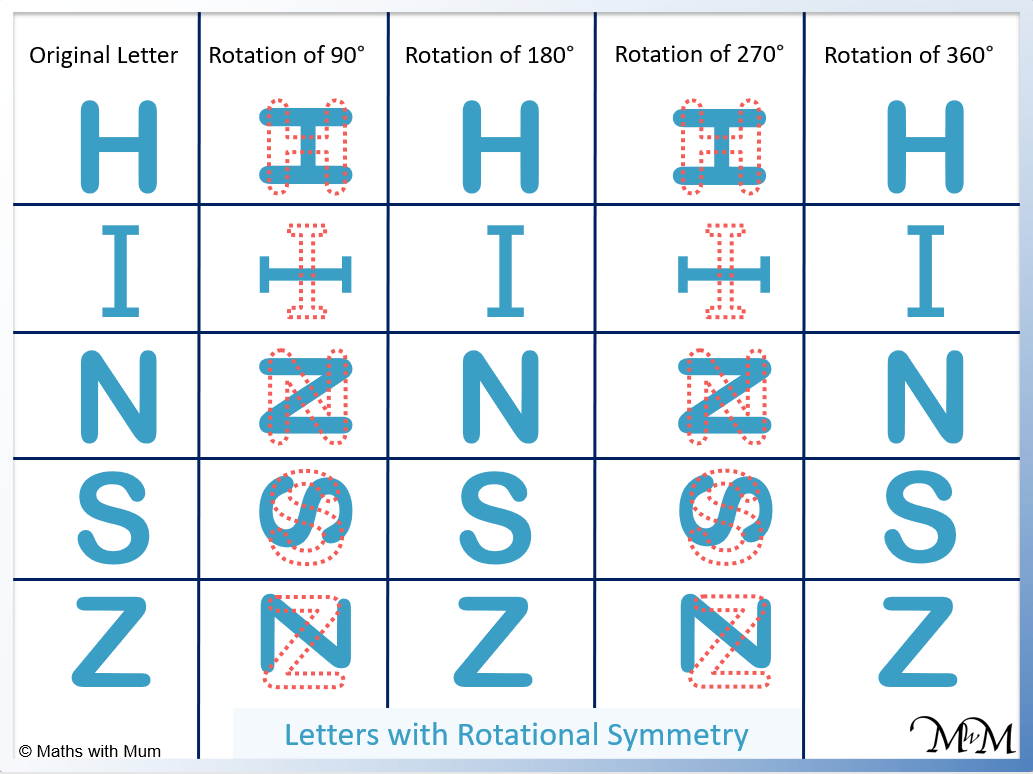Both of the capital letters O and X also have order of rotational symmetry 2.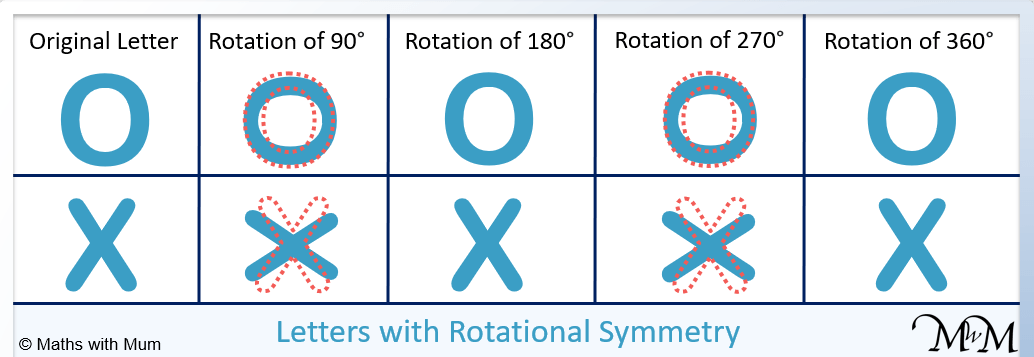It may look like X has a rotational symmetry of order 4, however, it is slightly longer than it is wide and it does not look the same when rotated 90 degrees.

The letter O is also longer than it is wide and does not look exactly the same when rotated 90 degrees.

## What is the Difference Between Rotational and Reflective Symmetry?

Rotational symmetry is how many times a shape fits into its original image when rotated a full turn. Reflective symmetry (or line symmetry) is the number of lines of symmetry pass through the centre of the shape so that both sides of the line look the same. The order of rotational symmetry is not the necessarily the same as the order of reflective symmetry.

In the triangle below we can see that the rotational symmetry is order 3 because the triangle can fit into its original shape 3 times when rotated. The reflective symmetry is also order 3 because there are 3 mirror lines of symmetry.In the kite below, the kite only fits into itself once and so, the order of rotational symmetry is 1.

There is one line of reflective symmetry going through the middle. Each side of this line looks identical.The order of rotational symmetry is not always the same as the order of reflective symmetry. For example in a trapezoid, there are no lines of symmetry and so the order of reflective symmetry is 0. However, the trapezoid fits into its outline once and so the order of rotational symmetry is 1.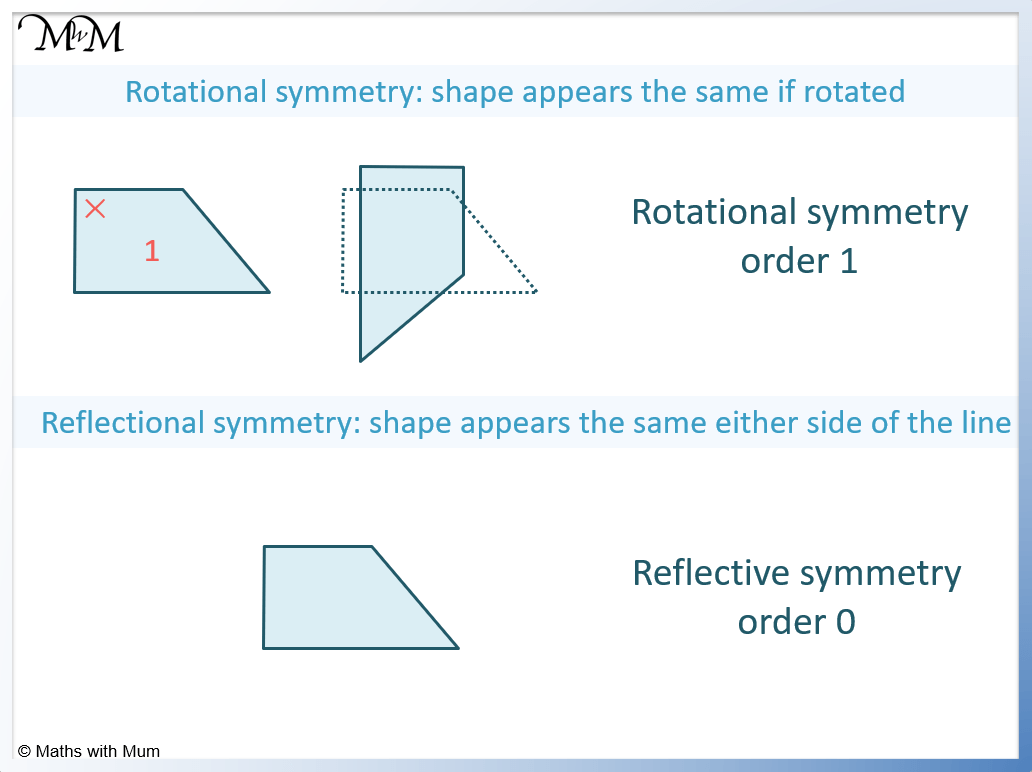The main difference between rotational symmetry and reflective symmetry is that a shape always has at least one degree of rotational symmetry when it fits into its own outline. A shape does not necessarily have any lines of reflective symmetry.

Here is a table showing the difference in rotational and reflective symmetry for several shapes.

Shape Order of Rotational Symmetry Order of Reflective Symmetry
Scalene Triangle 0 0
Isosceles Triangle 1 1
Equilateral Triangle 3 3
Rectangle 2 2
Square 4 4
Parallelogram 2 0
Rhombus 2 2
Trapezoid 1 0
Isosceles Trapezoid 1 1
Kite 1 1
Circle Infinite Infinite
Regular Pentagon 5 5
Regular Hexagon 6 6
Regular Heptagon 7 7
Regular Octagon 8 8
Regular Nonagon 9 9
Regular Decagon 10 10

## What is the Angle of Rotational Symmetry?

The angle of rotational symmetry is the smallest angle that a shape must be rotated to fit within its original outline. When rotated through this angle, it must look as though it has not been rotated at all. The angle of rotational symmetry can be calculated by dividing 360° by the order of rotational symmetry of the shape.

For example, a square has an angle of rotational symmetry of 90°. Rotating a square by 90° results in the square looking identical to before.The angle of rotational symmetry can also be found by dividing 360° by the order of rotational symmetry of the shape.

A square has a rotational symmetry order 4. This means that there are 4 positions that a square can be rotated into where it looks the same as before it was rotated.

360° ÷ 4 = 90° and so the angle of rotational symmetry is 90°.

Here is a list of the angle of rotational symmetry for various shapes:

Shape Order of Rotational Symmetry Angle of Rotational Symmetry
Equilateral Triangle 3 120°
Rectangle 2 180°
Square 4 90°
Parallelogram 2 180°
Rhombus 2 180°
Trapezoid 1 360°
Kite 1 360°
Regular Pentagon 5 72°
Regular Hexagon 6 60°
Regular Heptagon 7 51.4°
Regular Octagon 8 45°
Regular Nonagon 9 40°
Regular Decagon 10 36°

## Can a Shape have Rotational Symmetry of Order Zero?

No shape can have rotational symmetry of order zero. The smallest order of rotational symmetry a shape can have is 1. This is because all shapes must look the same when rotated a full turn to their original position. A shape with no rotational symmetry has rotational symmetry order 1.## Real Life Examples of Rotational Symmetry

Examples of rotational symmetry in real life include windmills, wheels, fidget spinners, fan blades, ferris wheels and the recycling logo.

Here is an example of a windmill fan showing rotational symmetry of order 3.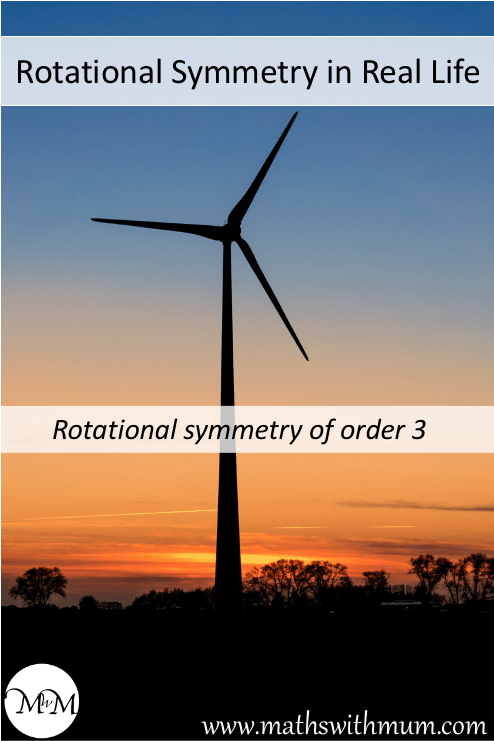Here is an example of a ferris wheel which looks the same whenever a new carriage reaches the top.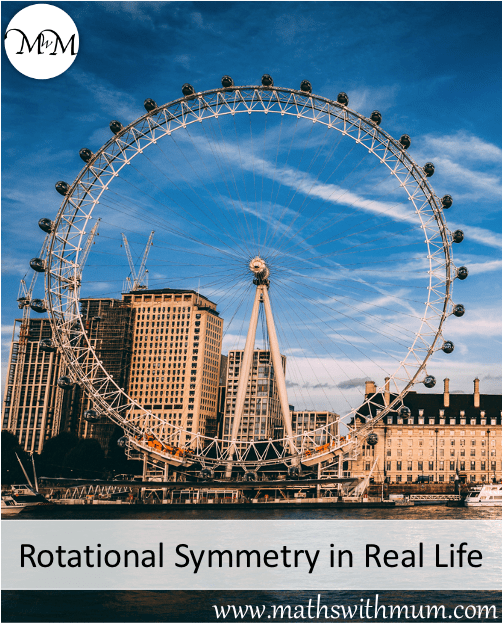## Rotational Symmetry in Nature

Examples of rotational symmetry in nature include flower petals, starfish, snowflakes and segments of fruit. These items will all look the same if rotated.

Here is an example of rotational symmetry in nature. This plant has four leaves that all look the same. The order of rotational symmetry is 4.Here is an example of a snowflake with order of rotational symmetry 6.A starfish is an example of rotational symmetry found in nature. It has rotational symmetry order 5 due to each of its 5 legs.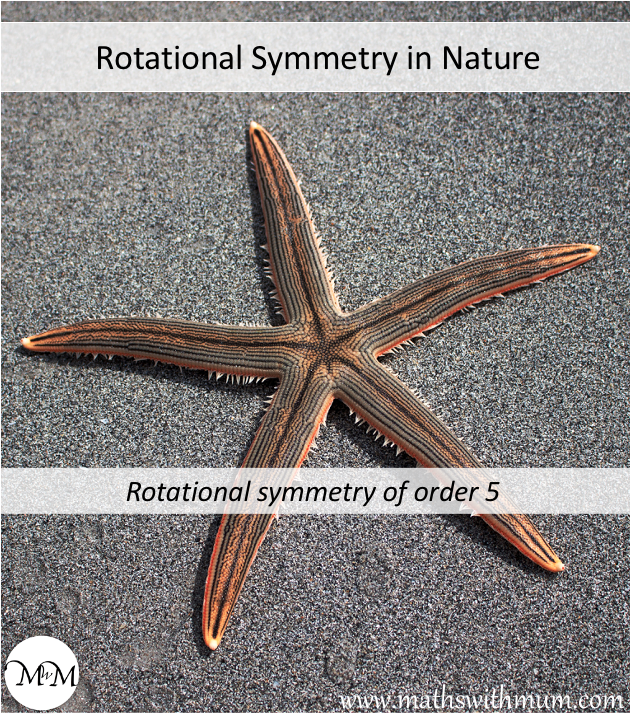Here is a fruit showing rotational symmetry. Rotating the cut piece of fruit, each segment looks the same.Here is a flower showing rotational symmetry.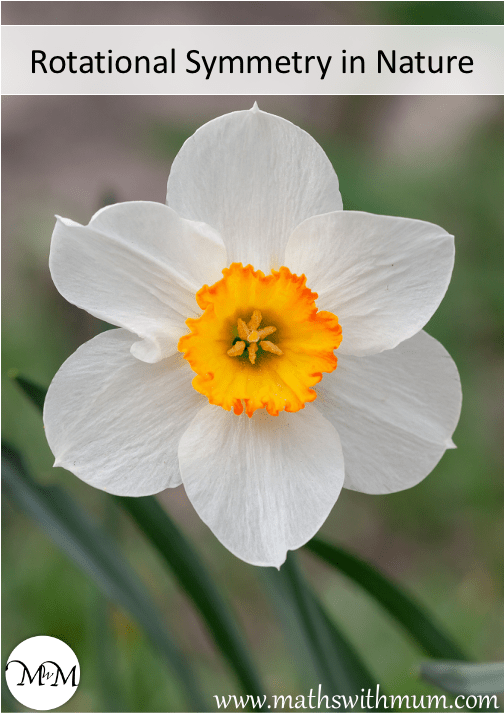## Examples of Rotational Symmetry

Here is a list of shapes and their order of rotational symmetry:

Shape Order of Rotational Symmetry
Scalene Triangle 1
Isosceles Triangle 1
Equilateral Triangle 3
Rectangle 2
Square 4
Parallelogram 2
Rhombus 2
Trapezoid 1
Kite 1
Circle Infinite
Regular Pentagon 5
Regular Hexagon 6
Regular Heptagon 7
Regular Octagon 8
Regular Nonagon 9
Regular Decagon 10

#### Rotational Symmetry of a Square

A square has rotational symmetry order 4. The angle of rotational symmetry is 90° since a square looks the same if it is rotated at 90°, 180°, 270° and 360°.#### Rotational Symmetry of a Rectangle

A rectangle has rotational symmetry order 2. The angle of rotational symetry is 180° since a rectangle looks the same if it is rotated at 180° and 360°.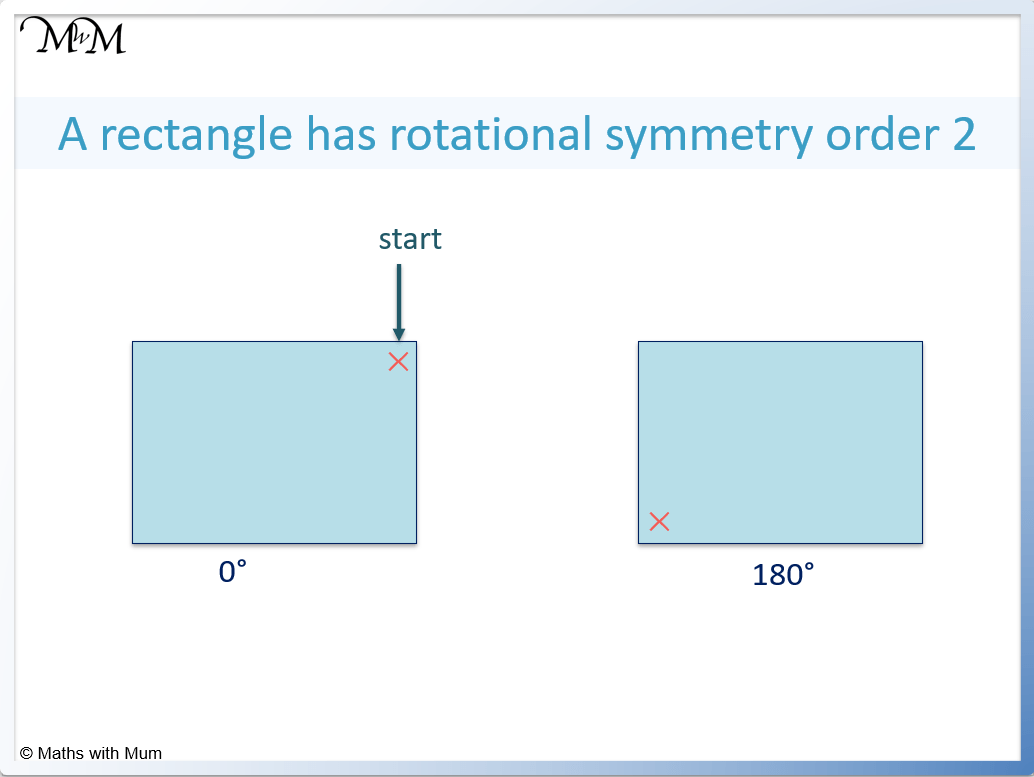#### Rotational Symmetry of a Parallelogram

A parallelogram has rotational symmetry order 2. The angle of rotational symetry is 180° since a parallelogram looks the same if it is rotated at 180° and 360°.#### Rotational Symmetry of a Rhombus

A rhombus has rotational symmetry order 2. The angle of rotational symetry is 180° since a rhombus looks the same if it is rotated at 180° and 360°.#### Rotational Symmetry of a Kite

A kite has no rotational symmetry. It only fits into its shape when it is not rotated. A kite has an order of rotational symmetry of 1.#### Rotational Symmetry of a Trapezoid

A trapezoid has no rotational symmetry. It only fits into its shape when it is not rotated. A trapezoid has an order of rotational symmetry of 1.

#### Rotational Symmetry of a Triangle

An equilateral triangle has a rotational symmetry of order 3. This means that it can fit into itself three times when rotated. All other triangles such as isosceles, scalene and right-angled triangles all have rotational symmetry of order 1.

Here is a table summarising the rotational symmetry of triangles:

Shape Order of Rotational Symmetry
Right-Angled Triangle 1
Scalene Triangle 1
Isosceles Triangle 1
Equilateral Triangle 3#### Rotational Symmetry of a Pentagon

A regular pentagon has rotational symmetry of order 5. This means that it looks the same in 5 different positions when rotated over a full turn. The angle of rotational symmetry of a regular pentagon is 72°. If a pentagon is not regular, it has no rotational symmetry.#### Rotational Symmetry of a Hexagon

A regular hexagon has rotational symmetry of order 6. This means that it looks the same in 6 different positions when rotated over a full turn. The angle of rotational symmetry of a regular hexagon is 60°.#### Rotational Symmetry of a Circle

A circle is the only shape that has infinite rotational symmetry. This means that it looks the same no matter what angle it is rotated. No other shape has this property. A circle is also the only shape that contains an infinite number of lines of reflective symmetry.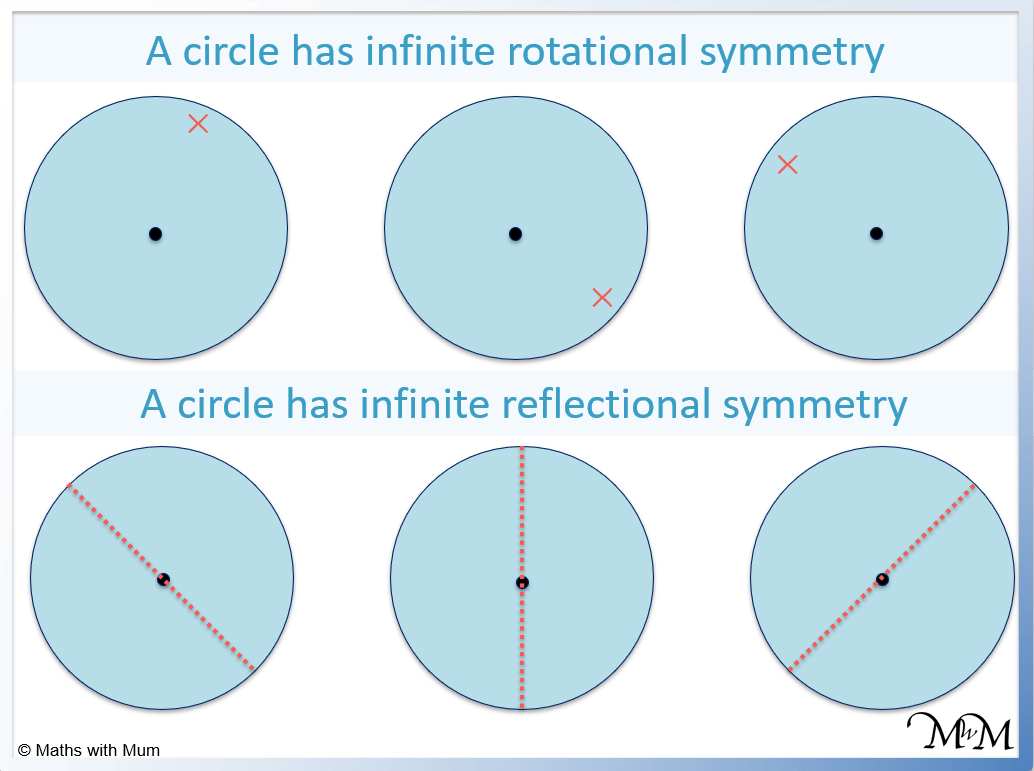A circle is the only shape that has rotational symmetry of infinite order and reflective symmetry of infinite order.

#### Rotational Symmetry of a Star

A star has the same rotational symmetry as the number of points it contains. For example, a 4-pointed star has rotational symmetry order 4 and a 5-pointed star has rotational symmetry order 5.

Here is a 4-pointed star. We can see that the star looks the same every 90 degrees.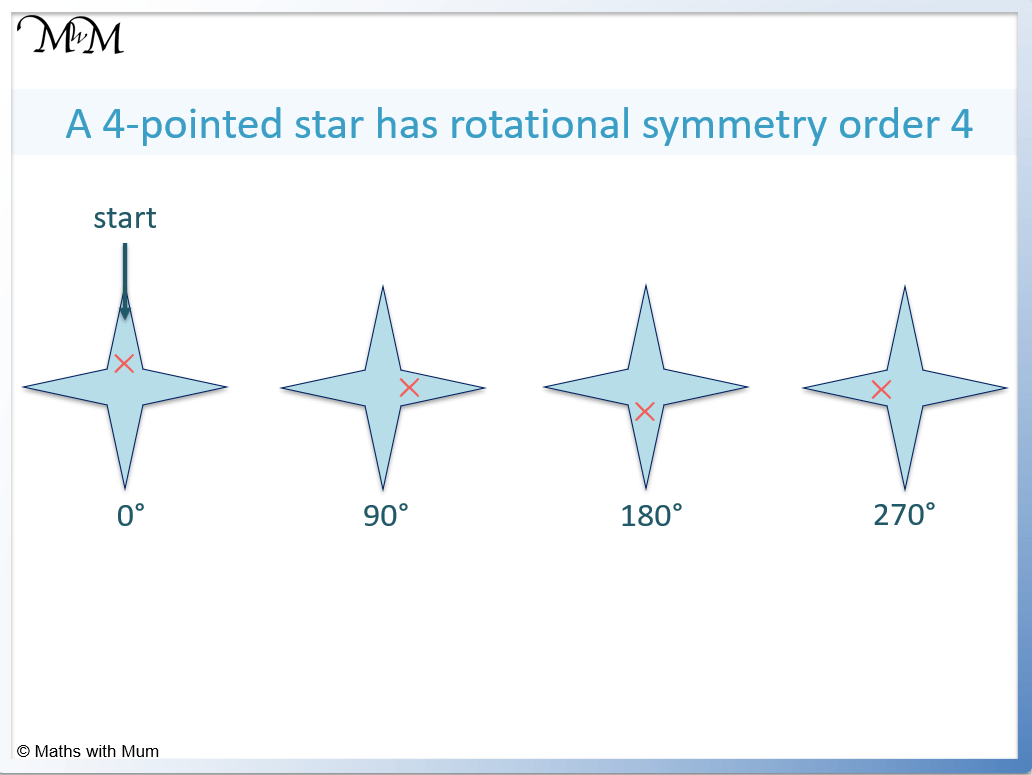Here is a 5-pointed star. It looks the same every rotation of 72 degrees.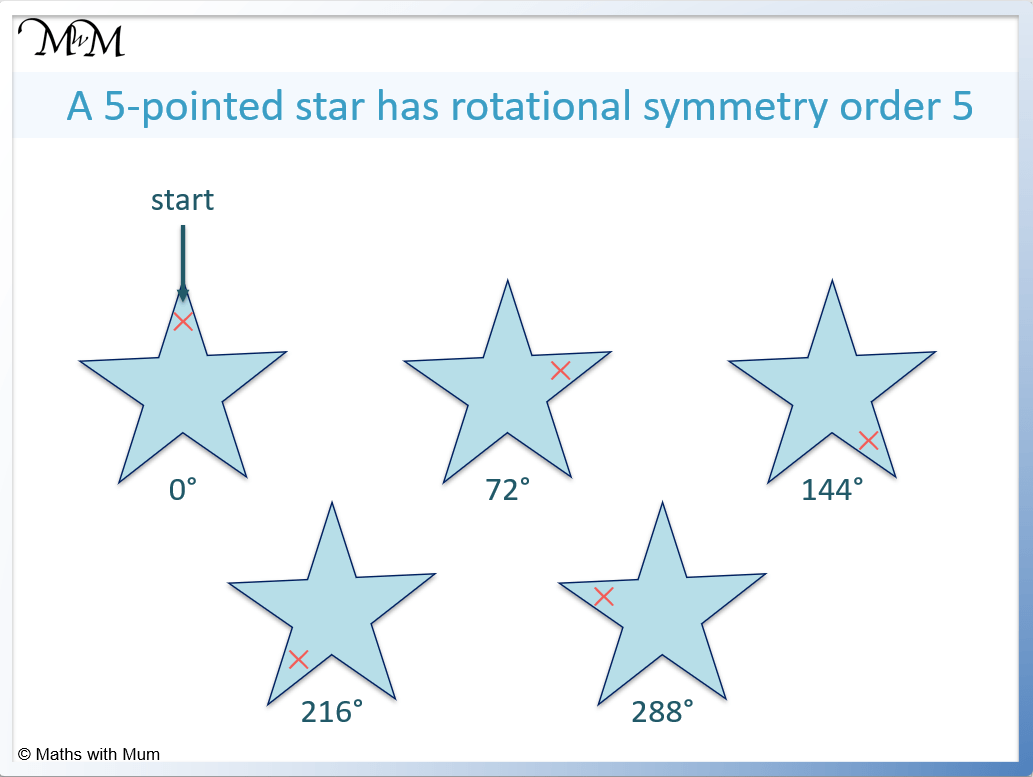#### Rotational Symmetry of a Regular Polygon

All regular polygons have a rotational symmetry equal to the number of sides. For example, an equilateral triangle has rotational symmetry of order 3, a square has rotational symmetry order 4 and a regular nonagon has rotational symmetry order 9.

A regular polygon is a shape with sides of equal length.

Simply count the number of sides of a regular polygon to find its order of rotational symmetry.

An equilateral triangle has rotational symmetry order 3.A square has rotational symmetry of order 4.A regular pentagon has rotational symmetry of order 5.## Shapes with Rotational Symmetry Order 1

If a shape has rotational symmetry order 1, then it has no rotational symmetry. If it is rotated then it will not look the same as its original image.Now try our lesson on Classifying Angles as Acute, Obtuse, Right or Reflex where we learn how to describe different angles.